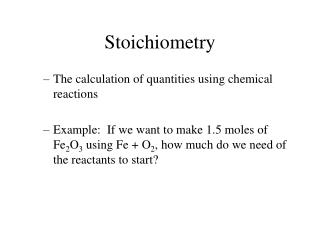Download PresentationStoichiometry

# Stoichiometry - PowerPoint PPT PresentationDownload Presentation## Stoichiometry

- - - - - - - - - - - - - - - - - - - - - - - - - - - E N D - - - - - - - - - - - - - - - - - - - - - - - - - - -
##### Presentation Transcript

1. Stoichiometry • The calculation of quantities using chemical reactions • Example: If we want to make 1.5 moles of Fe2O3 using Fe + O2, how much do we need of the reactants to start?

2. Mole Ratio • Conversion factor derived from the coefficients of a balanced equation. • It’s the BRIDGE to get across to other elements/compounds

3. N2 + 3H2 2 NH3 • 1 mole N2 / 3 mole H2 • 2 mole NH3 / 1 mole N2 • 3 mole H2 / 2 mole NH3

4. Mole-Mole Calculations • Predict products (possible) • Balance equation • Determine mole ratios • Start with what the problem gives you - ALWAYS! • Use the correct mole ratio

5. Magnesium and oxygen react to form ____________. • How many moles of the product are produced when 1.2 moles of oxygen react with excess magnesium? • How many moles of magnesium reacted in the problem above?